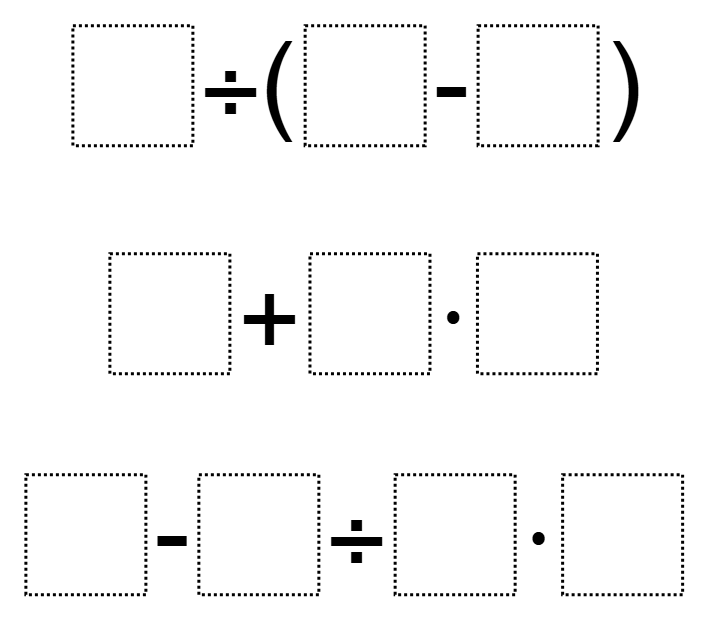# Order of Operations 5

Directions: Using the digits 0 to 9 at most one time each, place a digit in each box so that each expression is simplified to a different odd number.### Hint

How do we know which digits are easier to place?
How can we tell which operations might affect getting a whole number result for our expression?

There are many different solutions to this problem.

5 ÷ (8 – 7) = 5
9 + 0 x 6 = 9
3 – 4 / 2 ÷ 1 = 1

Source: Molly Rawding

## Add Fractions with Decimal Sums

Directions: Using the digits 1 to 9 at most one time each, place a digit …

1.Is the intent to use each number only once in the ENTIRE set?

2.3.what do we do on this

4.hey I’m like really confused!!!

5.On the second set shouldn’t the answer be 54? I thought that when I was in school the math was done in order except for brackets. So the question should look like 9+(0*6)=9

•I think you have to haeve an odd number and their are also many different answers for this.

6.HMMMMMMMMMMMMMMMMMMMMMMMMMM… Can we use decimals ¯\_(ツ)_/¯

7.I was forced to do this 🙁 so I feel like this rn ¯\_(ツ)_/¯

8.9.i am so confused

10.11.The possible solution given should end with multiplication in the equation, not division.

3 – 4 / 2 ÷ 1 = 1 should be 3 – 4 / 2 x 1 = 1

Not a big deal, just a typo.

12.My student and I got the following
7 / (6-5) =7
1 + 2 x 0 =1
9 – 8 / 4 x 3 =3

13.9 / (8-5) = 3
3 + 6 x 2 = 15 –> 3 + (6×2) = 15
7 – 4 / 1 x 0 = 7 —> 7-((4/1)x 0)) = 7

14.7 / ( 8 – 1 ) = 1

0 + 5 x 9 = 45

3 – 4 / 2 x 6 = 9

GOT A NEW ONE I THINK!!!

•Aleksandra Kowalczyk

YES CORRECT

15.I think you have to haeve an odd number and their are also many different answers for this.